Wednesday 22nd September 2021

CBSE Guess > Papers > Question Papers > Class XII > 2006 > Physics > Physics Compartment Delhi Set - I.

Physics — 2006 (Set I — Compartment Delhi)

Q. 1. Name the electromagnetic radiation to which the following wavelengths belong: 1

(a) 10-3m
(b) 1 A

Q. 2. Draw the graph showing the distribution of kinetic energy of electrons emitted during beta decay. 1

Q. 3. How will the photoelectric current change on decreasing the wavelength of incident radiation for a given photosensitive material? 1

Q. 4. How does the d.c. current gain of a transistor change, if the width of the base region is increased?

Q. 5. What is the length of a dipole antenna to transmit signals of frequency 200MHz? 1

Q. 6. An electric dipole of length 10cm having charges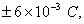placed at 300 with respect to a uniform electric field, experiences a torque of magnitude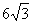Nm. Calculate

(i) the magnitude of the electric field.
(ii) the potential energy of the dipole. 2

Q. 7. A plane electromagnetic wave of frequency 25 MHz travels in free space along the x-direction. At a particular point in space and time the electric vector is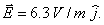Calculate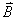at this point. 2

Q. 8. A conducting rod of length l is moved in a magnetic field of magnitude B with velocity v such that the arrangement is mutually perpendicular. Prove that the emf induced in the rod is | E I = B / v. 2

Or

A rectangular coil of area A, having number of turns N is at f revolutions per second in a uniform magnetic field B, the field being perpendicular to the coil Prove that the maximum emf induced in the coil is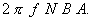. 2

Q. 9. A concave lens has the same radii of curvature for both sides and has a refractive index 1.6 in air. In the second case, it is immersed in a liquid of refractive index 1.4. Calculate the ratio of the focal lengths of the lens in the two cases. 2

Q. 10. ‘The output of an unregulated d.c. power supply is to be regulated. Name the device that can be used for this purpose and draw the relevant circuit diagram. 2

Q. 11. Derive an expression for the maximum force experienced by a straight conductor of length l, carrying current I and kept in a uniform magnetic field, B. 2

Q. 12. State two characteristics of nuclear force. Why does the binding energy per nucleon decrease with increase in mass number for heavy nuclei like 235U? 2

Q. 13. Define electric flux. Write its S.I. units. Using Gauss’ theorem, derive an expression for the electric field intensity at any point outside a charged spherical shell. 3

Q. 14. Derive the expression for the energy stored in a parallel plate capacitor with air between the plates. How does the stored energy change if air is replaced by a medium of dielectric constant K? 3

Q. 15. Define the term current density of a metallic conductor. Deduce the relation connecting current density (J) and the coductivity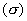of the conductor, when an electric field E, is applied to it. 3

Q. 16. Using Biot — Savart law derive the expression for the magnetic field at a distance x along the axis from the centre of a current carrying circular loop. 3

Q. 17. The height of a T.V. tower at a place is 400 m. Calculate

(i) the maximum range upto which signals can be received from time tower and
(ii) area covered by the transmission.
(Radius of the Earth 6400 km) 3

Q. 18. Explain the three modulation techniques employed for tansferring digital data into analog form, using diagrams. 3

Q. 19. In the following circuit, calculate, (I) the capacitance ‘C’ of the capacitor, if the power factor of the circuit is unity, and (ii) also calculate the Q-factor of the circuit 3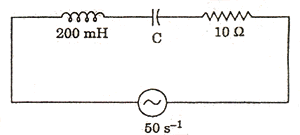Q. 20. Deduce an expression for the self-inductance of a long solenoid of N turns, having a core of relative permeability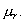3

Q. 21. Two wavelengths of sodium light 590 nm, 596 nm are used, in turn, to study the diffraction taking place at a single slit of aperture 2 x 10-6 m. The distance between the slit and the screen is 1.5 m. Calculate the separation between the positions of first maximum of the diffraction pattern obtained in the two cases. 3

Or

In Young’s slit experiment, interference fringes are observed on a screen, kept at D from the slits. If the screen is moved towards the slits by 5 x 10-2 m, the change in fringe width is found to be 3 x 10-5 m. If the separation between the slits is 10-3 m, calculate the wavelength of the light used. 3

Q. 22. Sketch the graphs, showing the variation of stopping poential with frequency of incident for two photosensitive materials A and B having thresh old frequencies V0 > V0' respectively.

(i) Which of the two metals, A or B has higher work function?
(ii) What information do you get from the slope of the graphs?
(iii) What does the value of the intercept of graph ‘A’ on the potential axis represent?3

Q. 23. Three identical specimens of magnetic materials Nickel, Antimony, Aluminium are kept in a non-uniform magnetic field. Draw the modification in the field lines in each case. Justify your answer. 3

Q. 24. Define the term decay constant of a radioactive nucleus.
Two nuclei P, Q have equal number of atoms at t = 0. Their half lives are 3 hours and 9 hours respectively. Compare their rates of disintegration, after 18 hours from the start. 3

Q. 25. State Kirchoff’s laws of an electrical network. Using Kirchoff’s laws, calculate the potential difference across the 8 ohm resistor.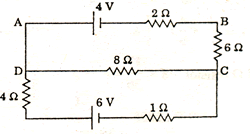State the working principle of a potentiometer. Explain, with the help of a circuit diagram, how the emf of two primary cells are compared by using a potentiometer.
In a potentiometer arrangement, a cell of emf 1.20 volt gives a balance point at 30 cm length of the wire. This cell is now replaced by another cell of unknown emf. If the ratio of the emfs of the two cells is 1.5, calculate the difference in the balancing length of the potentiometer wire in the two cases. 5

Q. 26. Draw a circuit diagram of a common-emitter amplifier. Deduce an expression for its voltage gain. Explain briefly how the output voltage is out of phase by 180° with the input voltage. 5

Or

Draw a circuit diagram to study the input and output characteristics of a n-p-n transistor in its common-emitter configuration. Draw the typical input and output characteristics and explain how these graphs are used to calculate (I) input resistance. (ii) output resistance and (iii) current amplification factor of the transistor.5

Q. 27. Derive the expression for the refractive index of the material of the prism in terms of the angle of the prism and angle of minimum deviation.

Use this formula to calculate the angle of minimum deviation for an equilateral triangular prism of refractive index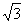Or

Draw a labelled ray diagram of an astronomical telescope for the near point adjustment. You are given three lenses of powers 0.5 D, 4 D, 10 D. State, with reason, which two lenses will you select for constructing a good astronomical telescope.
Calculate the resolving power of this telescope, assuming the diameter of the objective lens to be 6cm and the wavelength of light used to be 540 nm. 5

 Physics 2006 Question Papers Class XII Delhi Outside Delhi Compartment Delhi Compartment Outside DelhiSet ISet ISet ISet I

 CBSE 2006 Question Papers Class XIIEnglishSociologyFunctional EnglishPsychologyMathematicsPhilosopyPhysicsComputer ScienceChemistryEntrepreneurshipBiologyInformatics PracticesGeographyMultimedia & Web TechnologyEconomicsBiotechnologyBusiness StudiesPhysical EducationAccountancyFine ArtsPolitical ScienceHistoryAgriculture